## FPGA 之 SOPC 系列（七）NIOS II 高级技术_FPGA技术江湖的博客-程序员秘密7.1 定制基于Avalon的用户外设

7.2 定制Nios II用户指令

7.3 Nios II C语言至硬件加速编译器(C2H)简介7.1 定制基于Avalon的用户外设

+

NIOS II是一个建立在FPGA上的嵌入式软核处理器，除了可以根据需要任意添加已经提供的外设外，用户还可以通过定制用户逻辑外设和定制用户指令来实现各种应用要求。

（1）指定硬件功能

（2）指定微处理器访问和控制该硬件的应用程序接口

（3）定义一个AVALON接口：提供正确的控制机制、足够的吞吐性能

（4）采用VHDL或Verilog编写硬件设计

（5）单独测试硬件设计

（6）编写C头文件，定义寄存器映射

（7）使用元件编辑器将硬件和软件文件打包成一个元件

（8）例化元件为SOPC系统的一个模块

（9）使用NIOSII处理器测试元件的寄存器级访问

（10）编写元件的驱动程序

（11）反复改进元件的设计：硬件、软件、元件更新

（12）编译完整的包含一个或多个该元件的SOPC系统

（13）执行系统级的验证，若必要，进行反复设计

（14）完成元件设计，发布共享元件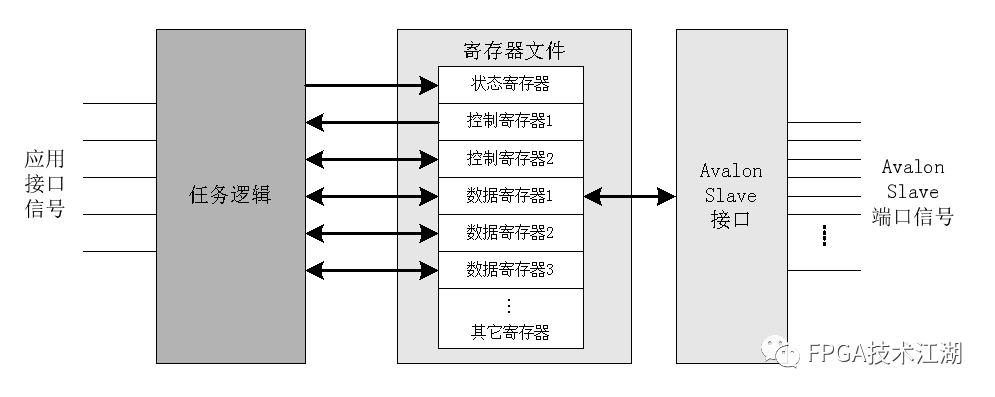LED灯亮度原理：

PWM输出一个占空比可调的方波。当一个周期11个时钟，高电平输出7个时钟时的PWM输出波形如下图所示。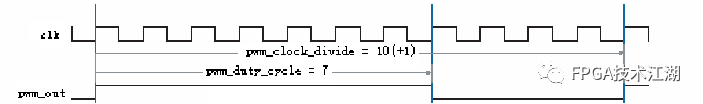PWM输出波形

PWM设计说明：

1．任务逻辑按一个简单时钟进行同步操作。

2．可以使用微控制器（Nios II）来设置PWM的周期和占空比的值。因此要提供一个可对PWM寄存器进行读写的接口和控制逻辑。

3．定义寄存器来存储PWM周期和占空比的值。

4．微控制器可以通过控制寄存器的禁止位来关闭PWM输出。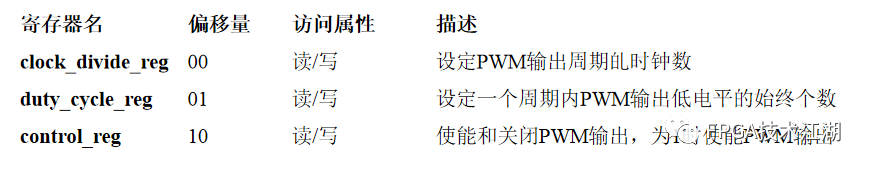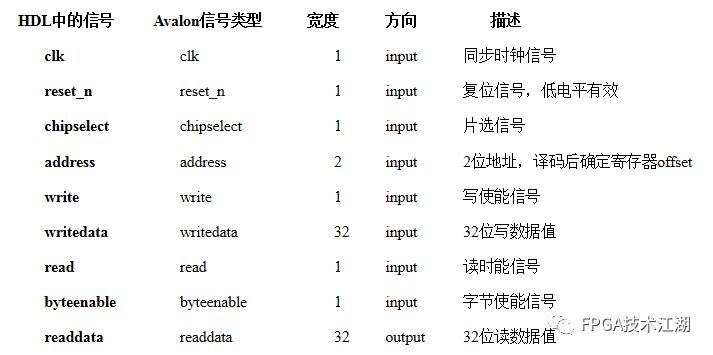``module led_control(``    clk,``    reset_n,``    chipselect,``    address,``    write,``    writedata,``    read,``    byteenable,``    readdata,``    led_out``    );``  input  clk;``  input  reset_n;``  input  chipselect;``  input  [1:0]address;``  input  write;``  input  [31:0]  writedata;``  input  read;``  input  [3:0]  byteenable;``  output  [31:0]  readdata;``  output  led_out;``   ``  reg    [31:0]  clock_divide_reg;``  reg    [31:0]  duty_cycle_reg;``  reg    control_reg;``  reg    duty_cycle_reg_selected;``  reg    clock_divide_reg_selected;``  reg    control_reg_selected;``  reg    [31:0]  led_counter;``  reg    [31:0]  readdata;``  reg    led_out;``  wire  led_enable;``  ``  //地址译码``  always  @  (address)``    begin``            clock_divide_reg_selected<=0;``            duty_cycle_reg_selected<=0;``            control_reg_selected<=0;``        case(address)``            2'b00:clock_divide_reg_selected<=1;``            2'b01:duty_cycle_reg_selected<=1;``            2'b10:control_reg_selected<=1;``        default:``            begin``              clock_divide_reg_selected<=0;``              duty_cycle_reg_selected<=0;``              control_reg_selected<=0;``            end``        endcase``     end``  //写led输出周期的时钟数寄存器``  always @ (posedge clk or negedge reset_n)``  begin``    if(reset_n==1'b0)``      clock_divide_reg=0;``    else``    begin``      if(write & chipselect & clock_divide_reg_selected)``        begin``          if(byteenable)``            clock_divide_reg[7:0]=writedata[7:0];``          if(byteenable)``            clock_divide_reg[15:8]=writedata[15:8];``          if(byteenable)``            clock_divide_reg[23:16]=writedata[23:16];``          if(byteenable)``            clock_divide_reg[31:24]=writedata[31:24];``        end``    end``  end``//写led周期占空比寄存器``always @ (posedge clk or negedge reset_n)``begin``  if(reset_n==1'b0)``    duty_cycle_reg=0;``  else``  begin``    if(write & chipselect & duty_cycle_reg_selected)``      begin``        if(byteenable)``          duty_cycle_reg[7:0]=writedata[7:0];``        if(byteenable)``          duty_cycle_reg[15:8]=writedata[15:8];``        if(byteenable)``          duty_cycle_reg[23:16]=writedata[23:16];``        if(byteenable)``          duty_cycle_reg[31:24]=writedata[31:24];``      end``  end``end``//写控制寄存器``always @ (posedge clk or negedge reset_n)``begin``  if(reset_n==1'b0)``    control_reg=0;``  else``  begin``    if(write & chipselect & control_reg_selected)``      begin``        if(byteenable)``          control_reg=writedata;``      end``  end``end``//读寄存器``always @ (address or read or clock_divide_reg or duty_cycle_reg or control_reg or chipselect)``begin``  if (read & chipselect)``    case(address)``      2'b00:readdata<=clock_divide_reg;``      2'b01:readdata<=duty_cycle_reg;``      2'b10:readdata<=control_reg;``      default:readdata=32'h8888;``    endcase``end``//控制寄存器``assign led_enable=control_reg;``//led功能部分``always @(posedge clk or negedge reset_n)``begin``  if(reset_n==1'b0)``    led_counter=0;``  else``  begin``    if(led_enable)``    begin``      if(led_counter>=clock_divide_reg)``        led_counter<=0;``      else``        led_counter<=led_counter+1;``    end``    else``      led_counter<=0;``  end``end``always @(posedge clk or negedge reset_n)``begin``  if(reset_n==1'b0)``    led_out<=1'b0;``  else``  begin``    if(led_enable)``    begin``      if(led_counter<=duty_cycle_reg)``        led_out<=1'b1;``      else``        led_out<=1'b0;``    end``    else``      led_out<=1'b0;``  end``end`` ``endmodule``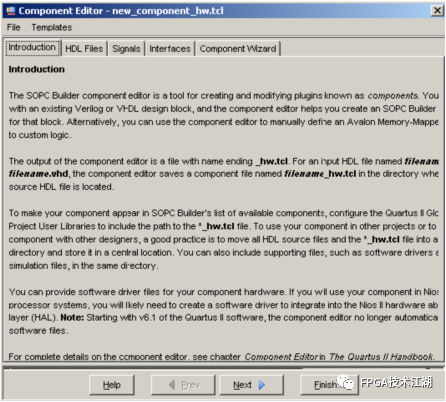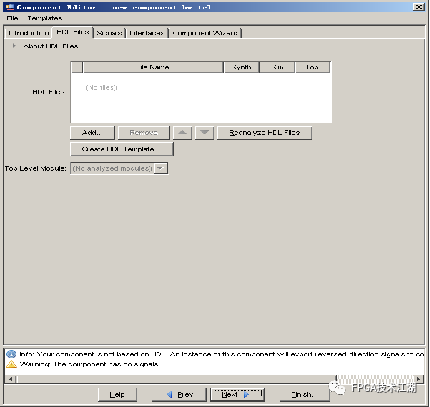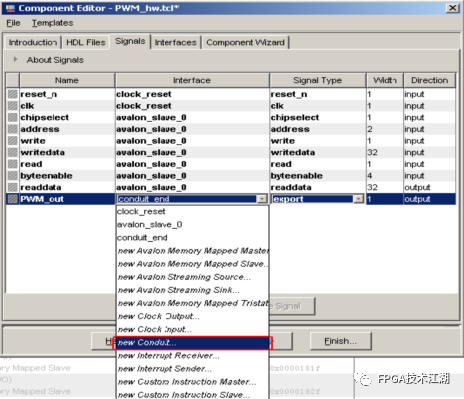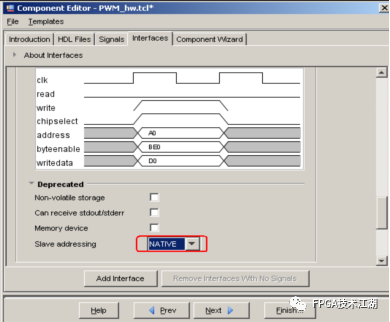• 自动地址分配

• 分配中断

• 管脚分配

• 编译

````#include<unistd.h>``#include"system.h"``//````typedef struct{
````                    volatile unsigned int divi;``                    volatile unsigned int duty;``                    volatile unsigned int enable;``                }LED_CONTROL;   ``int main()````{
````    int dir=1;``    //``    LED_CONTROL *led_control=(LED_CONTROL*)LED_CONTROL_BASE;``    ``    //``    led_control->divi=1000;``    led_control->duty=0;``    led_control->enable=1;``    ````    while(1){
``````        if(dir>0){
````            if(led_control->duty<led_control->divi)``                led_control->duty+=100;``            else``                dir=0;``            }````            else{
````                if(led_control->duty>0)``                    led_control->duty-=100;``                else``                    dir=1;``            }``            usleep(100000);``        }   ``        ``        return 0;``}           ````

7.2 定制Nios II用户指令

+

1）NIOSII处理器配置向导提供了图形化界面添加封装用户定制指令；

2）NIOSII支持256条定制指令；

3）NIOSII IDE在system.h中为每条定制指令产生一个宏，用户在应用程序中通过调用宏访问定制指令。

Nios II定制指令综述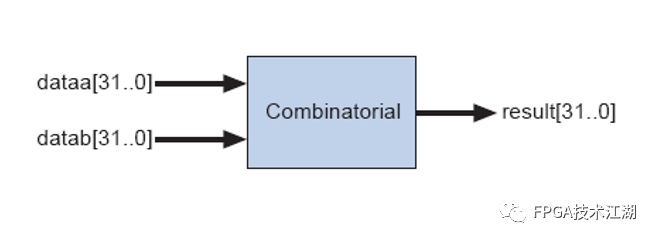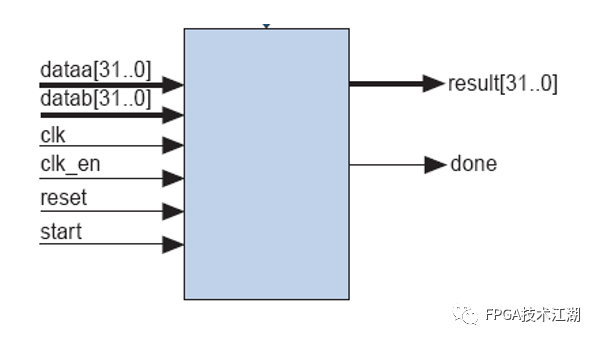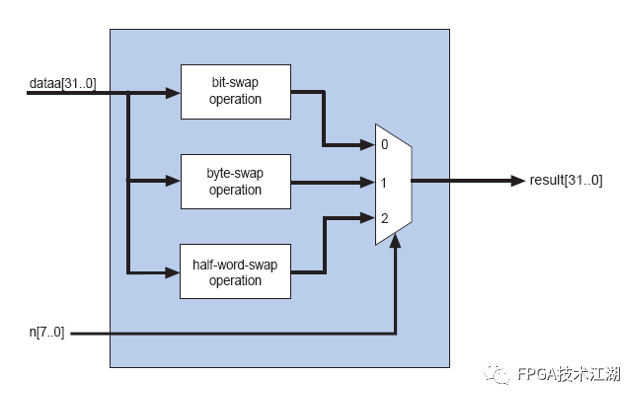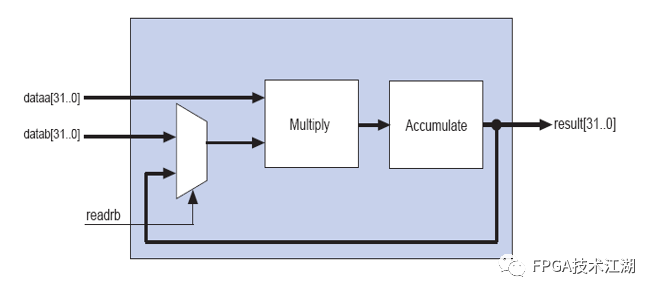1．导入HDL文件实现定制指令；

2．通过DSP Builder实现定制指令加速模块；

3．直接使用SOPC Builder中自带的定制指令。

（1）打开NIOSII CPU的定制指令设置页；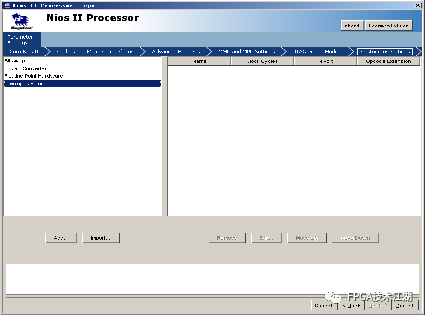（2）添加用户定制指令设计文件；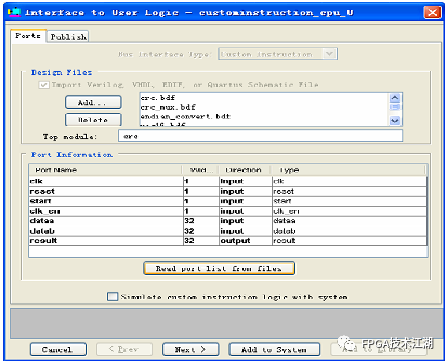（3）发布用户定制指令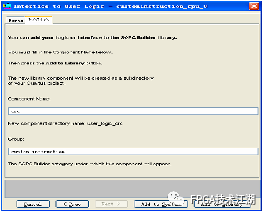（4）将定制指令加入系统，完成定制指令添加。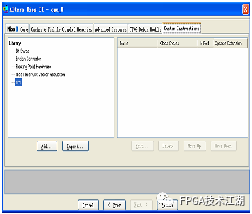7.3 Nios II C语言至硬件加速编译器(C2H)简介

+

• C2H是能够提升对时间性能要求较高的ANSIC函数的工具，它将这些函数转换为FPGA中的硬件加速器。

• C2H支持标准ANSI C代码，可加速实现多种应用程序，提高其运行效率，包括访问片内、外部存储器和外设等。

• C2H帮助Nios II用户以最少的资源占用来达到提高系统性能的目的。

Nios II C2H编译器设计流程非常简单，编写好应用程序后，用户需要做的工作首先是分析软件代码，确定出现性能瓶颈的函数，然后在Nios II IDE中高亮显示所需的函数，右键单击加速便可以生成自动链接至软件流程的硬件加速器。FPGA 之 SOPC 系列第七篇就到这里结束，下一篇将带来第八篇，程序固化相关内容。各位大侠，明天见！

### 2021年第四届“中青杯”A题 汽车组装车间流水线物料配送问题（超详细思路及代码）_车间行车送料算法_KeepLearners的博客-程序员秘密

2021年第四届“中青杯”A题 汽车组装车间流水线物料配送问题（超详细思路及代码）题目背景：汽车制造冲压、焊接、涂装和总装四大关键工艺。总装过程中物料的配送补充，是本次题目的核心。物料补充工作称为运输，一般由拖车完成。拖车接收到任务指令后，需要进行如下操作：1）取料：前往目标物料的存储位，将物料装载至拖车；2）配送：将物料运送至目标工位和卸载。需要注意的是，在不超过小车容量的情况下，多个任务可以同时进行。问题 1：某总装车间内有两条并行的流水线，其布局如图1所示，其道路关键节点和工作点见附件1。该车

### 《Spring Security3》第四章第三部分翻译上（配置安全的密码）_weixin_33804990的博客-程序员秘密

2019独角兽企业重金招聘Python工程师标准&gt;&gt;&gt; ...